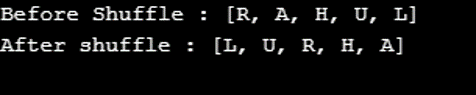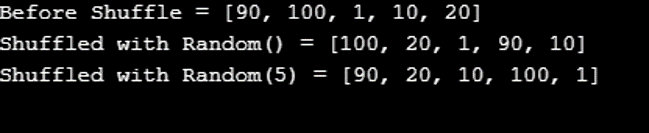Introduction to Shuffle() in Java

Java has many built-in functions to perform different operations on collections or other data types and one of them is shuffle. Shuffle function is available in many other languages like Python.

Shuffle function is used to shuffle the collection elements. It randomly permutes the list elements passed in parameters. There are two methods to shuffle in Java one is using the collections shuffle method and another is by using random class. Collection shuffle function can also be called in two ways one with a random parameter to specify randomness and another without parameter. shuffle(<list>) shuffle(<list>, <random function>) You need to pass the list so that we convert the array to list then pass it to collection.shuffle and again convert the result to an array. Syntax for Shuffle() in Java

Declaration for shuffle method:

public static void shuffle(List<?> list)

public static void shuffle(List<?> list, Random random)

Parameters:

List: The list which you will pass will be shuffled. Random: It’s the random function passed with seed value which will become the source of randomness.

Returns:

Shuffle function doesn’t return any value, just shuffles the list. Examples of Shuffle() in Java

In the example below, we created a list from an array with some alphabets and used the shuffle method to shuffle the array. Every time you run you would get a different shuffled list.

Example #1

Code:

import java.util.*; public class CollectionsShuffleExampleWithoutRandom { public static void main(String[] args) { List<String> list = Arrays.asList(“R”, “A”, “H”, “U”, “L”); System.out.println(“Before Shuffle : “+list); Collections.shuffle(list); System.out.println(“After shuffle : “+list); } }

Output:Example #2

In the example below, we are creating a linked list of integers and adding some integers to it. But here we passed another argument that is the Random function which will become the source of Randomness. Then we passed Random function with seed value 5. This is another flavor or we can say the method of using shuffle function with Randomness.

Code:

import java.util.*; public class CollectionsShuffleWithRandom { public static void main(String[] args) { //Create linked list object LinkedList<Integer> list = new LinkedList<Integer>(); //Add values list.add(90); list.add(100); list.add(1); list.add(10); list.add(20); System.out.println(“Before Shuffle = “+list); //Random() to shuffle the given list. Collections.shuffle(list, new Random()); System.out.println(“Shuffled with Random() = “+list); //Random(5) to shuffle the given list. Collections.shuffle(list, new Random(5)); System.out.println(“Shuffled with Random(5) = “+list); } }

Output:Shuffling without Shuffle Method

If you want more control over shuffle then you could write your own method to shuffle the list with the random method and another approach to shuffle the list. This method is more flexible and easy to fit in any application. You can actually understand how shuffle works inside Java’s built-in method.

Input: An int array

Output: Shuffled array(in a randomized order)

Example:

public static int[] ShuffleArray(int[] array){ Random rand = new Random();  // Random value generator for (int i=0; i<array.length; i++) { int randomIndex = rand.nextInt(array.length); int temp = array[i]; array[i] = array[randomIndex]; array[randomIndex] = temp; } return array; }

The above function where you just need to pass an array integer and it will return a shuffled array. Inside the function, you can see we are iterating the array till its length and generating a random number and it will be treated as array index which will be swapped with another array. This is how the elements will be swapped inside an array. The resulting array will be a swapped one.

From the above function, we can get a basic concept of the shuffle function where a list of values will be sent and a random number will be generated each time while iterating the elements in the array. The element will be swapped with another element in the same list with the index randomly generated from a random function.

Important Points for Shuffle Function This method works on randomly permuting the list elements and shuffling them. Each time executed the result can be different. The function doesn’t take much time and runs in linear time. If you provide a list that doesn’t implement the RandomAccess interface, then shuffle will first copy the list into an array, then will shuffle the array copy, and then copies back it into a list which is the result and return it. Shuffle traverses the list backward – the last element up to the second element repeatedly. While traversing it randomly selects elements and swap with the current position. The randomly selected element is from the portion of the list from the first element to the current element.

Exceptions:

UnsupportedOperationException: if the passed list or list-iterator does not support a set operation. Applications of Shuffle

There could be many situations where the shuffle function below are some applications:

Shuffling a list of questions in a QA application. Shuffling list of quotes that you want to show to your app users every day. Lottery application where you want to assign a ticket number to the user. Generating unique transaction numbers for a payment field. Generation unique user id for different users can be prefixed to user id. It can also be used in cryptographic applications. Conclusion

In the above article, we understood how shuffle works and how you can use it. There can be multiple use cases somewhere you would be using shuffle function with random parameter else without random parameter and some applications might need a different flexible implementation where you can write your own shuffle function using Java’s Random function.

Recommended Articles

This is a guide to Shuffle() in Java. Here we discuss the Introduction and Important Points for Shuffle Function along with different examples and its code implementation. You may also have a look at the following articles to learn more –

The post Shuffle() in Java appeared first on EDUCBA.